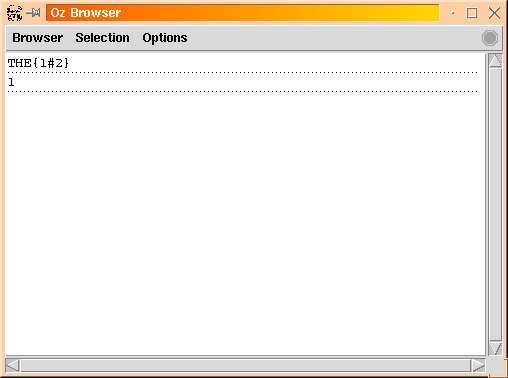3.2 Simple Example

As a simple example, lets consider a minuscule English lexicon consisting of the words: a, the, man, men and just the information about whether the word is singular or plural. We are going to encode `singular' as the integer 1 and `plural' as the integer 2.

declare
Sing = 1
Plur = 2
Lexicon = o(a   : [Sing]
the : [Sing Plur]
man : [Sing]
men : [Plur])

Now, we can represent the ``number'' information of a word W by introducing a FD variable I and constraining its domain using the information in the lexicon. For example for an occurrence of article the, we could write:

declare THE
THE::Lexicon.the

We can observe this variable in the browser [Pop99] by invoking {Browse THE}, and we see:I.e. THE is bound to a FD variable whose domain just contains the integers 1 and 2. We can do the same for an occurrence of the word man:

declare MAN
MAN::Lexicon.man
{Browse MAN}

and we see:Finally we can impose the constraint that the determiner must agree with the noun, i.e. THE=MAN, and the display is updated as follows:The ambiguous number information of the determiner has been disambiguated.

Denys Duchier
Version 1.2.0 (20010221)